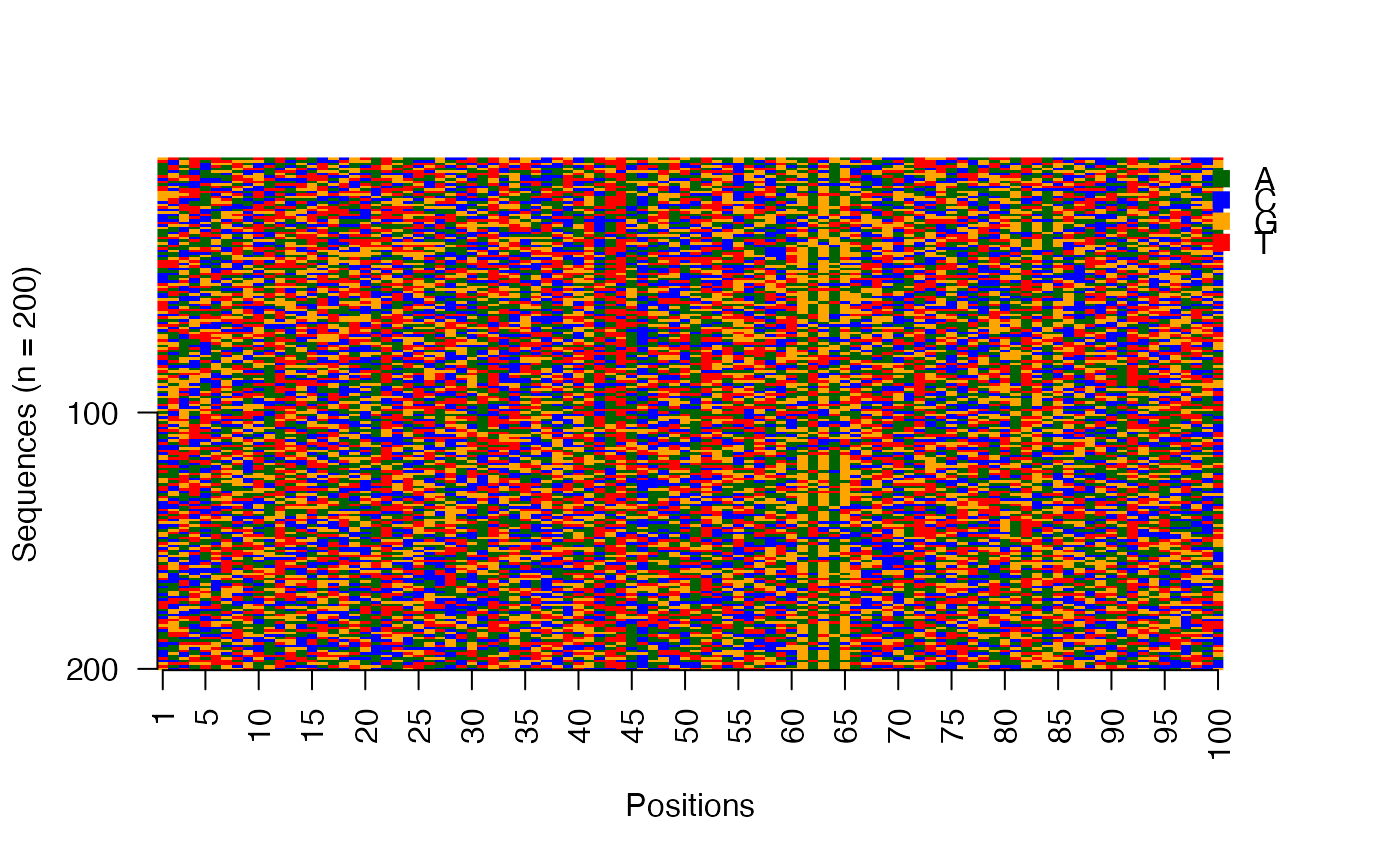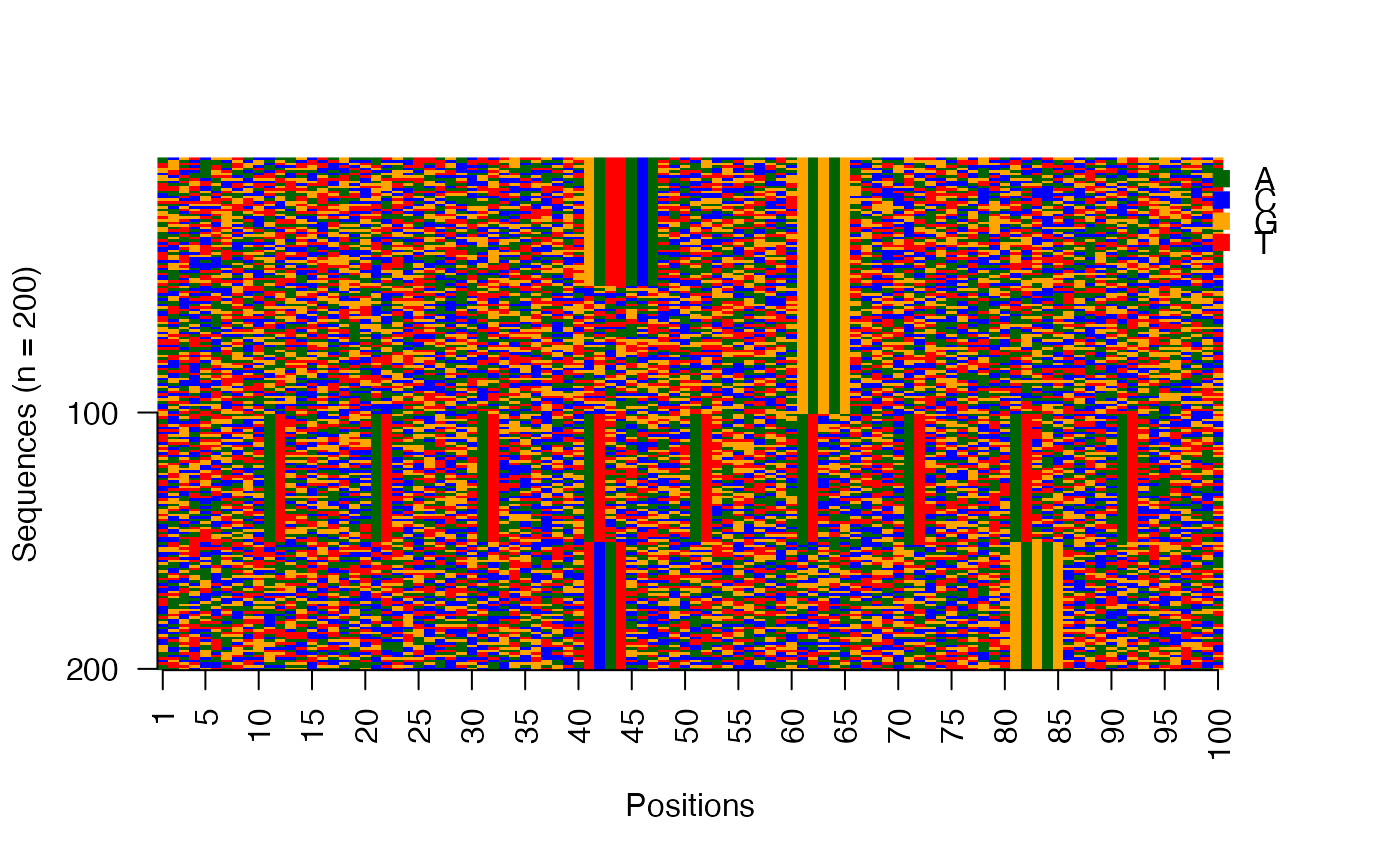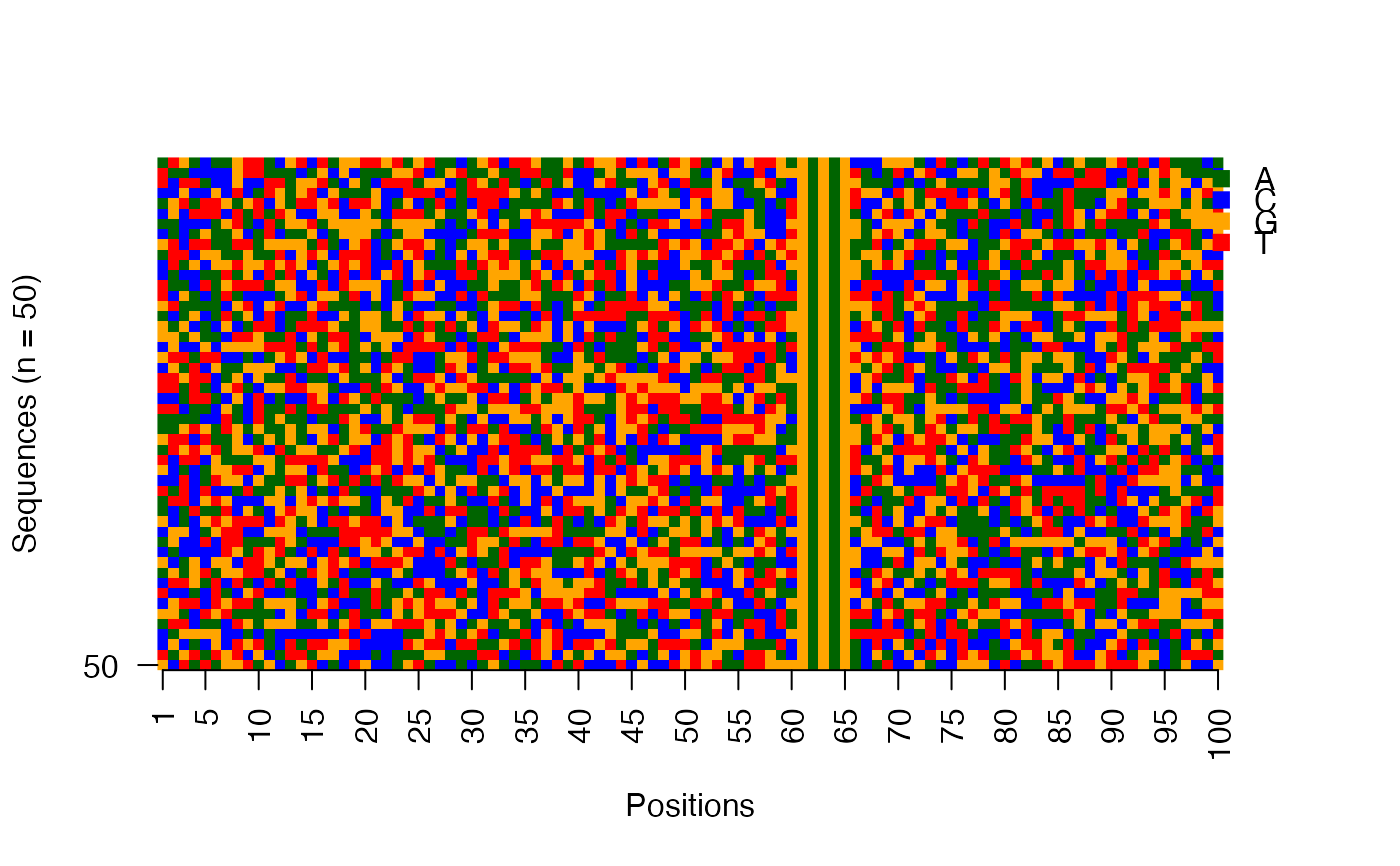This function plots the collection of sequences as an image matrix.

viz_seqs_acgt_mat(
seqs,
pos_lab = NULL,
xt_freq = min(length(pos_lab), 5),
yt_freq = min(length(seqs), 100),
use_col = c("darkgreen", "blue", "orange", "red"),
use_legend = Biostrings::DNA_BASES,
save_fname = NULL,
file_type = "PNG",
f_width = 450,
f_height = 900,
f_units = "px"
)

## Arguments

seqs

The sequences as a DNAStringSet object.

pos_lab

The labels to be used for the sequence positions. Default: Sequence positions are labeled from 1 to the length of the sequences.

xt_freq

The x-axis tick frequency. Expects a positive integer less than the length of the sequences. Default is 5.

yt_freq

The y-axis tick frequency. Expects a positive integer less than number of sequences. Default is 100.

use_col

A vector of four colors used for the DNA bases A, C, G, and T (in that order).

Logical. Whether legend should be added to the plot. Default is TRUE.

use_legend

A character vector of letters in the input sequences. Default is DNA_BASES, used for DNA sequences.

save_fname

Specify the filename (with extension) for saving the plot to disk.

file_type

Specify the file type, namely PNG, JPEG, TIFF.

f_width

Specify the width for the plot. This depends on the length of sequences.

f_height

Specify the height for the plot. This depends on the number of sequences.

f_units

Specify the units in which the height and width are given.

## Value

Nothing returned to the R interpreter.

Other visualization functions: viz_bas_vec(), viz_pwm()

## Examples


package = "seqArchR", mustWork = TRUE))

# Image matrix of sequences in the input order
viz_seqs_acgt_mat(seqs = seqs_str(res))# Image matrix of sequences ordered by the clustering from seqArchR
use_seqs <- seqs_str(res, iter = NULL, cl = NULL, ord = TRUE)
viz_seqs_acgt_mat(seqs = use_seqs)# Image matrix of sequences belonging to a single cluster
use_seqs <- seqs_str(res, iter = 2, cl = 2)
viz_seqs_acgt_mat(seqs = use_seqs)